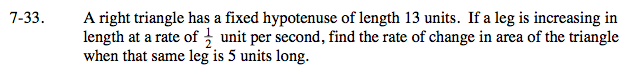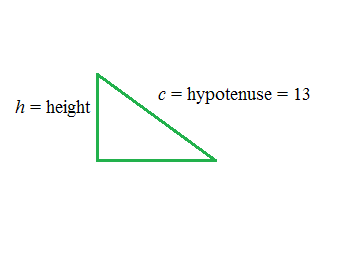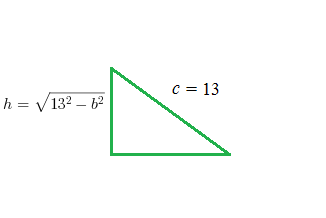### Home > CALC > Chapter 7 > Lesson 7.1.3 > Problem7-33

7-33.

A right triangle has a fixed hypotenuse of length 13 units. If a leg is increasing in length at a rate ofunit per second, find the rate of change in area of the triangle when that same leg is 5 units long. Homework Help ✎Sketch the triangle and label the known information.

$\text{So start with an area equation: }A=\frac{1}{2}bh.$

Notice that there are two variables. It would be easier to have only one.

Use the Pythagorean Theorem to find the length of the height in terms of b.
Then rewrite the function with only one variable:

$A=\frac{1}{2}(b)(\sqrt{13^{2}-b^{2}})$the rate that the area is changing.

Substitute in all values that you know, including the specific base length, 5.